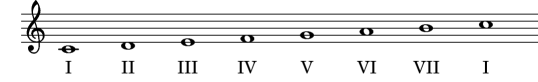Violin HomeMusic DictionaryScale Degrees

# Scale Degrees

Names and symbols used in harmonic analysis to denote tones of the scale as roots of chords. The most important are degrees I = tonic (T), IV = subdominant (S) and V = dominant (D).• The first scale degree is also known as the tonic
• The second scale degree is also known as the supertonic
• The fourth scale degree is also known as the subdominant
• The fifth scale degree is also known as the dominant
• The sixth scale degree is also known as the submediant
• The seventh scale degree is also known as the subtonic

 © 2014 multiple domain web hosting by TheViolinSite.com